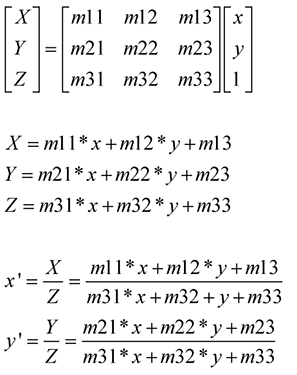# 【Computer Vision】图像单应性变换/投影/仿射/透视

1. projective transformation  = homography = collineation.

2. 齐次坐标：使用N+1维坐标来表示N维坐标，例如在2D笛卡尔坐标系中加上额外变量w来形成2D齐次坐标系$(x,y) \Rightarrow (x,y,w)$

3. 单应性变换是对齐次坐标下点的线性变换，可以通过矩阵运算来表达$x^{'}= Hx$H为非奇异矩阵.

二、基础变换

$$x' = \begin{pmatrix} R&t \\ 0^T&1\end{pmatrix}x$$

R为2*2旋转矩阵，t为2维列向量，0^T为0的二维行向量

$$x' = \begin{pmatrix} sR & t \\ 0^T & 1 \end{pmatrix}x$$

s为缩放尺度

$$x' = \begin{pmatrix}A&t \\ 0^T&1\end{pmatrix}x$$

A为2*2的非奇异矩阵,可被分解为如下: $A = R(\theta)R(-\phi)DR(\phi)$

$\lambda_1 和 \lambda_2$可以看做两个方向的缩放比.

$$x' = \begin{pmatrix}A&t \\ V^T&v\end{pmatrix}x$$python版本:2.7

1. 使用仿射矩阵进行image warping: 图像扭曲from scipy import ndimage
from numpy import *
from PIL import Image
from pylab import *
im = array(Image.open('trees_001.jpg').convert('L'))
H = array([[1.4,0.05,-100],[0.05,1.5,-100],[0,0,1]])
#im2  = ndimage.affine_transform(im,H[:2,:2],(H[0,2],H[1,2]))
im2  = ndimage.affine_transform(im,H[:2,:2],(H[0,2],H[1,2]))
figure()
gray()
imshow(im2)
show()

结果:posted @ 2017-08-10 10:07  VincentCheng  阅读(10223)  评论(0编辑  收藏  举报